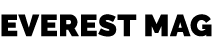News Trend TV

Trend Hub

## Categories

### Everest NewsUt interdum risus felis, eget rhoncus sem aliquam nec. Sed eu congue arcu. Duis ultricies orci nec diam malesuada accumsan. Aliquam pulvinar pulvinar orci, nec ornare ex efficitur ac. Proin quis laoreet quam. Praesent sagittis mollis turpis tempus sodales. Ut efficitur tortor nec condimentum ornare.

## A Detailed Look at The Area of The Rhombus

Rhombuses have the shape of diamonds. Rhombuses are subsets of parallelograms, and they all have equal sides, but opposite sides are parallel. They are equal in size and have opposite sides that are parallel. Squares have all right angles while rhombuses need not have right angles. A rhombus with right angles becomes a square, therefore. Rhombuses that do not have right angles are not squares.

They are quadrilaterals with two sets of parallel sides, equal opposite sides, and equal opposite angles – they are called equilateral quadrilaterals because all their sides are the same length. They are in many things around us, including kites, windows, rhombus earrings, buildings, diamonds, mirrors, and even sections of baseball fields. They have several properties, and the area of rhombus formula is widely used to solve various problems.

## Properties of a Rhombus

• All sides of a rhombus are equal i.e., AB=BC=DC=DA.
• Diagonals bisect each other at 90∘.i.e., diagonals BD and AC bisect each other at 90∘.
• Opposite sides are parallel in a rhombus i.e., AB∥CD and AD∥BC
• Opposite angles are equal in a rhombus i.e., ∠A=∠C,∠B=∠D.
• Adjacent angles add up to 180∘, i.e., ∠A+∠B=180∘, ∠B+∠C=180∘, ∠C+∠D=180∘, ∠A+∠D=180∘

## In What Ways Can the Area of the Rhombus Be Calculated?

A rhombus’ area is defined as the total area it occupies in a 2D space. Three methods can be used to calculate the area of the rhombus.

• Area of a rhombus with diagonals
• Area of a rhombus with side
• Area of rhombus using trigonometry concept

### How to Calculate the Area of a Rhombus Using Diagonals?

Imagine a rhombus PQRS, which has two diagonals, i.e. PR & QS. The following steps will help in finding the area:

• Calculate the length of diagonal 1, d1. It is the distance between P &R. When the diagonals of a rhombus intersect at the center, they form 4 right triangles perpendicular to each other.
• To find the length of diagonal 2, which is the distance between Q & S, we will need to find d2.
• Multiply both the diagonals, d1, and d2.
• The result should be divided by 2

Example: Calculate the area of a rhombus having diagonals equal to 5 cm and 7 cm.

Ans: Given that,

Diagonal 1, d1 = 5 cm

Diagonal 2, d2 = 7 cm

Area of a rhombus, A = (d1 × d2) / 2

= (5 × 7) / 2

= 35 / 2

= 17.5 cm 2

Hence, the area of the rhombus is 17.5cm 2

### How to Calculate the Area of a Rhombus Using Base & Height?

• To determine the base and height of a rhombus, choose one of its sides as the base, and the height of the rhombus is the altitude, which is the distance from the chosen base to the opposite side.
• Multiply the base and calculated height.

Example: Calculate the area of a rhombus if its base is 20 cm and height is 17 cm.

Ans: Given,

Base, b = 20 cm

Height, h = 17 cm

Area, A = b × h

= 20 × 17cm 2

A = 340cm 2

### How to Calculate the Area of a Rhombus Using Trigonometry?

• A squared length can be found by squaring any side.
• You can determine the Sine of one angle by multiplying it by the length.

Example: Calculate the area of a rhombus if the length of its side is 4 cm and one of its angle A is 30 degrees.

Ans: Given,

Side = s = 4 cm

Angle A = 30 degrees

Square of side = 4 × 4= 16

Area, A = s 2 × sin (30)

A = 16 × ½

A = 8 cm 2

You can learn more about rhombus, by taking guidance from Cuemath, one of the most favored and loved online maths teaching platforms.